# Diprotic Acids and Equivalence Points

aqion
aqion
May. 28, 2016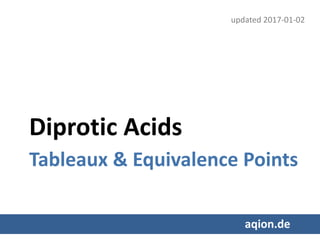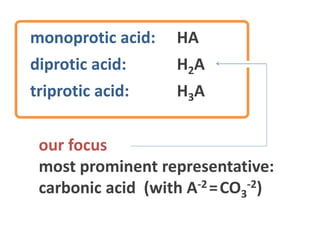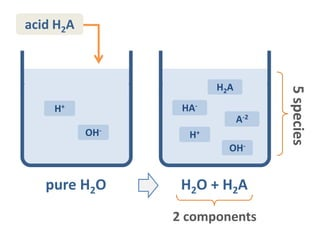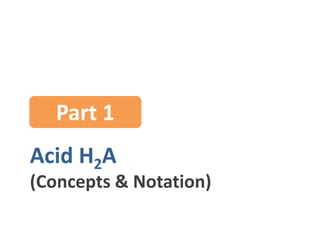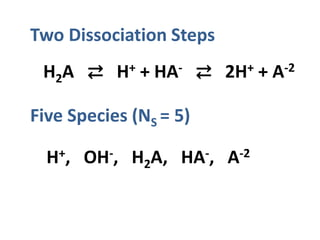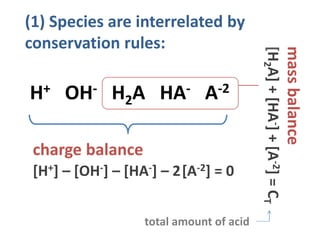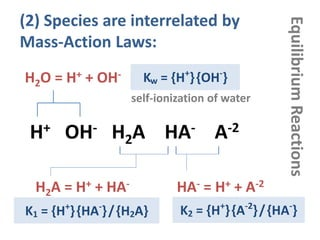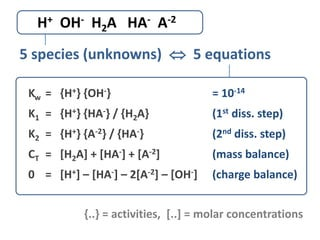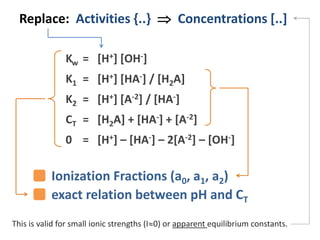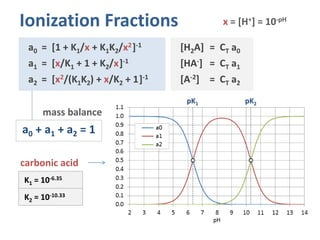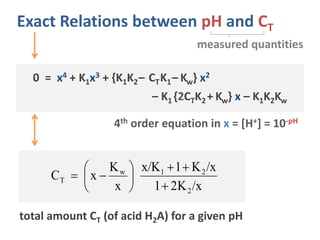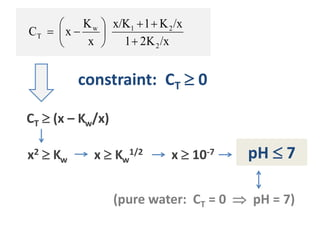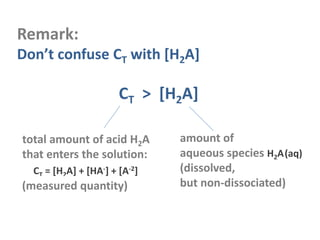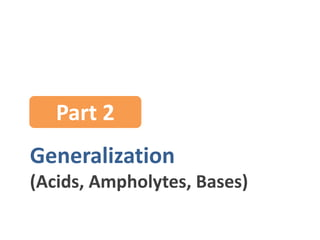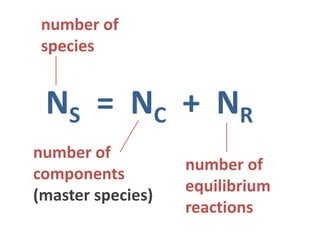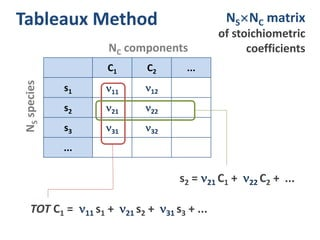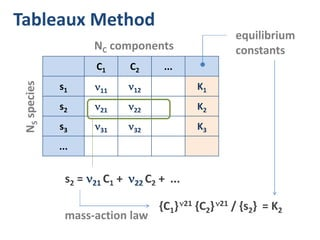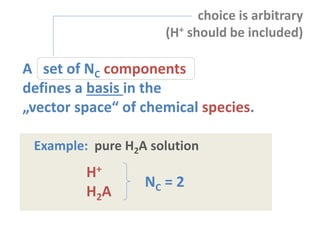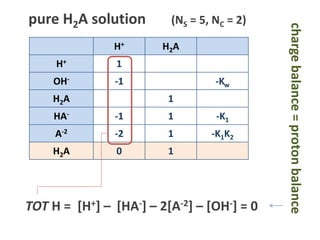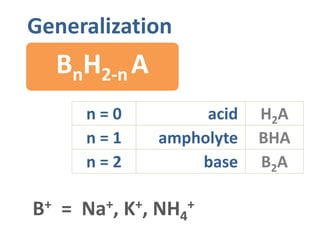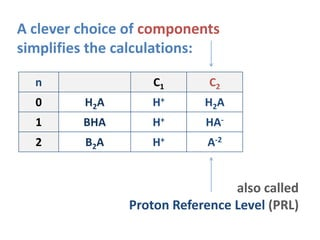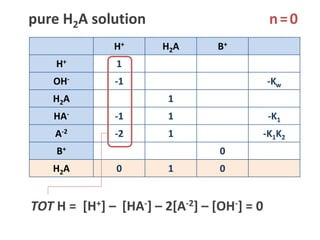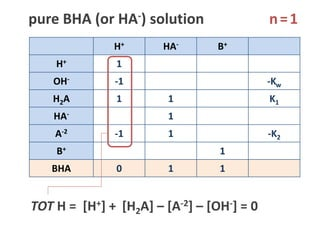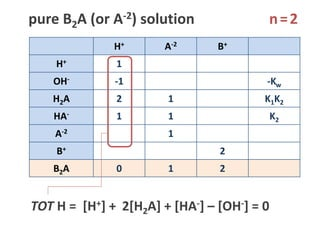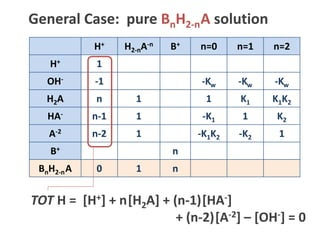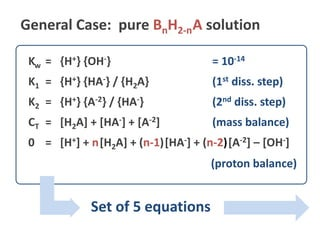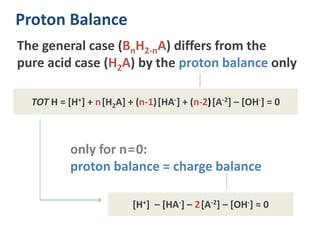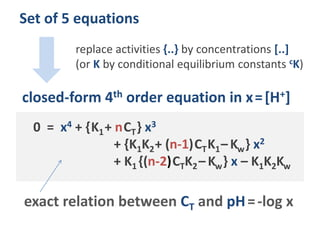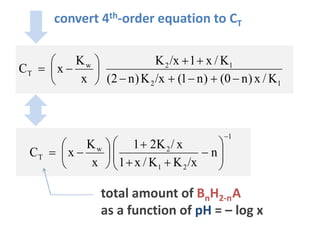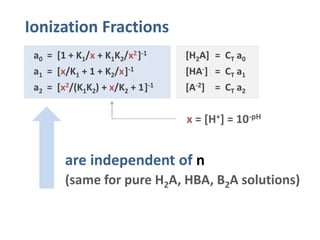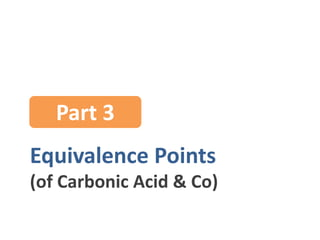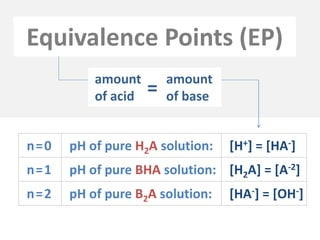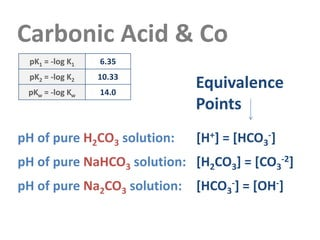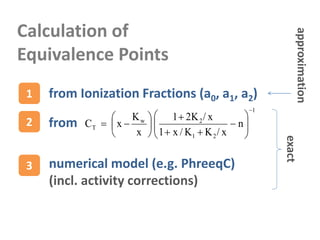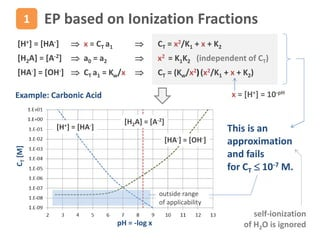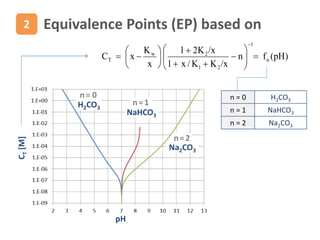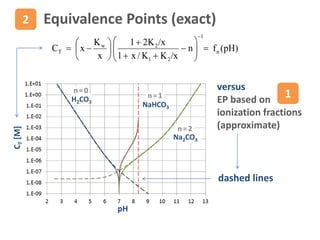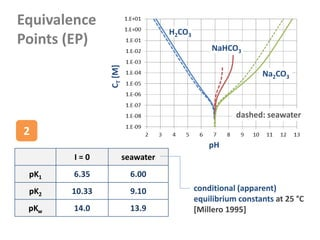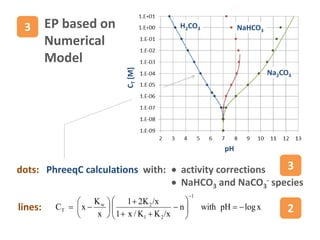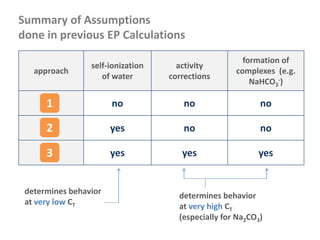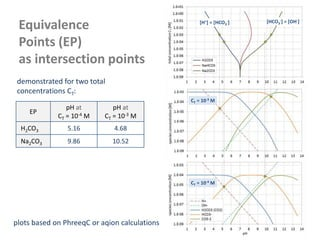1 of 42

### Diprotic Acids and Equivalence Points

1. Diprotic Acids Tableaux & Equivalence Points aqion.de updated 2017-04-15
2. monoprotic acid: HA diprotic acid: H2A triprotic acid: H3A our focus most prominent representative: carbonic acid (with A-2 =CO3 -2)
3. pure H2O OH- H+ H2O + H2A H2A HA- A-2 OH- H+ acid H2A 5species 2 components
4. Acid H2A (Concepts & Notation) Part 1
5. Two Dissociation Steps H2A ⇄ H+ + HA- ⇄ 2H+ + A-2 Five Species (NS = 5) H+, OH-, H2A, HA-, A-2
6. (1) Species are interrelated by conservation rules: H+ OH- H2A HA- A-2 charge balance massbalance [H2A]+[HA-]+[A-2]=CT [H+] – [OH-] – [HA-] – 2[A-2] = 0 total amount of acid
7. (2) Species are interrelated by Mass-Action Laws: H2A = H+ + HA- HA- = H+ + A-2 H2O = H+ + OH- Kw = {H+ }{OH- } K1 = {H+ }{HA- }/{H2A} H+ OH- H2A HA- A-2 K2 = {H+ }{A-2 }/{HA- } EquilibriumReactions self-ionization of water
8. H+ OH- H2A HA- A-2 Kw = {H+} {OH-} = 10-14 K1 = {H+} {HA-} / {H2A} (1st diss. step) K2 = {H+} {A-2} / {HA-} (2nd diss. step) CT = [H2A] + [HA-] + [A-2] (mass balance) 0 = [H+] – [HA-] – 2[A-2] – [OH-] (charge balance) 5 species (unknowns)  5 equations {..} = activities, [..] = molar concentrations
9. Replace: Activities {..}  Concentrations [..] Kw = [H+] [OH-] K1 = [H+] [HA-] / [H2A] K2 = [H+] [A-2] / [HA-] CT = [H2A] + [HA-] + [A-2] 0 = [H+] – [HA-] – 2[A-2] – [OH-] This is valid for small ionic strengths (I0) or apparent equilibrium constants. Ionization Fractions (a0, a1, a2) exact relation between pH and CT
10. Ionization Fractions a0 = [1 + K1/x + K1K2/x2]-1 [H2A] = CT a0 a1 = [x/K1 + 1 + K2/x]-1 [HA-] = CT a1 a2 = [x2/(K1K2) + x/K2 + 1]-1 [A-2] = CT a2 x = [H+] = 10-pH K1 = 10-6.35 K2 = 10-10.33 carbonic acid mass balance a0 + a1 + a2 = 1 pK1 pK2
11. Exact Relations between pH and CT 4th order equation in x = [H+] = 10-pH 0 = x4 + K1x3 + {K1K2– CTK1– Kw} x2 – K1 {2CTK2 + Kw} x – K1K2Kw x/K21 x/K1K/x x K xC 2 21w T          total amount CT (of acid H2A) for a given pH measured quantities
12. x/K21 x/K1K/x x K xC 2 21w T          constraint: CT  0 CT  (x – Kw/x) x2  Kw x  Kw 1/2 x  10-7 pH  7 (pure water: CT = 0  pH = 7)
13. Remark: Don’t confuse CT with [H2A] CT > [H2A] total amount of acid H2A that enters the solution: CT = [H2A] + [HA-] + [A-2] amount of aqueous species H2A(aq) (dissolved, but non-dissociated)(measured quantity)
14. Generalization (Acids, Ampholytes, Bases) Part 2
15. NS = NC + NR number of species number of equilibrium reactions number of components (master species)
16. C1 C2 ... s1 11 12 s2 21 22 s3 31 32 ... Tableaux Method TOT C1 = 11 s1 + 21 s2 +  31 s3 + ... NSNC matrix of stoichiometric coefficientsNC components NSspecies s2 = 21 C1 + 22 C2 +  ...
17. C1 C2 ... s1 11 12 K1 s2 21 22 K2 s3 31 32 K3 ... Tableaux Method {C1}21 {C2}21 / {s2} = K2 NC components NSspecies s2 = 21 C1 + 22 C2 +  ... equilibrium constants mass-action law
18. Example: pure H2A solution choice is arbitrary (H+ should be included) H+ H2A NC = 2 A set of NC components defines a basis in the „vector space“ of chemical species.
19. H+ H2A H+ 1 OH- -1 -Kw H2A 1 HA- -1 1 -K1 A-2 -2 1 -K1K2 H2A 0 1 pure H2A solution (NS = 5, NC = 2) TOT H = [H+] –  [HA-] – 2[A-2] – [OH-] = 0 chargebalance=protonbalance
20. Generalization BnH2-n A B+ = Na+, K+, NH4 + n = 0 acid n = 1 ampholyte n = 2 base H2A BHA B2A
21. A clever choice of components simplifies the calculations: n C1 C2 0 H2A H+ H2A 1 BHA H+ HA- 2 B2A H+ A-2 also called Proton Reference Level (PRL)
22. H+ H2A B+ H+ 1 OH- -1 -Kw H2A 1 HA- -1 1 -K1 A-2 -2 1 -K1K2 B+ 0 H2A 0 1 0 pure H2A solution TOT H = [H+] –  [HA-] – 2[A-2] – [OH-] = 0 n=0
23. H+ HA- B+ H+ 1 OH- -1 -Kw H2A 1 1 K1 HA- 1 A-2 -1 1 -K2 B+ 1 BHA 0 1 1 pure BHA (or HA-) solution TOT H = [H+] +  [H2A] – [A-2] – [OH-] = 0 n=1
24. H+ A-2 B+ H+ 1 OH- -1 -Kw H2A 2 1 K1K2 HA- 1 1 K2 A-2 1 B+ 2 B2A 0 1 2 pure B2A (or A-2) solution TOT H = [H+] + 2[H2A] + [HA-] – [OH-] = 0 n=2
25. H+ H2-nA-n B+ n=0 n=1 n=2 H+ 1 OH- -1 -Kw -Kw -Kw H2A n 1 1 K1 K1K2 HA- n-1 1 -K1 1 K2 A-2 n-2 1 -K1K2 -K2 1 B+ n BnH2-nA 0 1 n General Case: pure BnH2-nA solution TOT H = [H+] – [OH-] + n[H2A] + (n-1)[HA-] + (n-2)[A-2] = 0
26. Kw = {H+} {OH-} = 10-14 K1 = {H+} {HA-} / {H2A} (1st diss. step) K2 = {H+} {A-2} / {HA-} (2nd diss. step) CT = [H2A] + [HA-] + [A-2] (mass balance) 0 = [H+] + n[H2A] + (n-1)[HA-] + (n-2)[A-2] – [OH-] General Case: pure BnH2-nA solution (proton balance) Set of 5 equations
27. only for n=0: proton balance = charge balance The general case (BnH2-nA) differs from the pure acid case (H2A) by the proton balance only TOT H = [H+] + n[H2A] + (n-1)[HA-] + (n-2)[A-2] – [OH-] = 0 [H+] – [HA-] – 2[A-2] – [OH-] = 0 Proton Balance
28. Set of 5 equations replace activities {..} by concentrations [..] (or K by conditional equilibrium constants cK) closed-form 4th order equation in x=[H+] 0 = x4 + {K1+ nCT} x3 + {K1K2+ (n-1)CTK1– Kw} x2 + K1 {(n-2)CTK2– Kw} x – K1K2Kw exact relation between CT and pH=-log x
29. C C convert 4th-order equation to CT 12 12w T K/x)n0()n1(x/K)n2( K/x1x/K x K xC          total amount of BnH2-nA as a function of pH = – log x 1 21 2w T n x/KK/x1 x/K21 x K xC                 
30. Ionization Fractions a0 = [1 + K1/x + K1K2/x2]-1 [H2A] = CT a0 a1 = [x/K1 + 1 + K2/x]-1 [HA-] = CT a1 a2 = [x2/(K1K2) + x/K2 + 1]-1 [A-2] = CT a2 x = [H+] = 10-pH are independent of n (same for pure H2A, HBA, B2A solutions)
31. Equivalence Points (of Carbonic Acid & Co) Part 3
32. Equivalence Points (EP) n=0 pH of pure H2A solution: [H+] = [HA-] n=1 pH of pure BHA solution: [H2A] = [A-2] n=2 pH of pure B2A solution: [HA-] = [OH-] amount of acid amount of base=
33. Carbonic Acid & Co pH of pure H2CO3 solution: [H+] = [HCO3 -] pH of pure NaHCO3 solution: [H2CO3] = [CO3 -2] pH of pure Na2CO3 solution: [HCO3 -] = [OH-] Equivalence Points pK1 = -log K1 6.35 pK2 = -log K2 10.33 pKw = -log Kw 14.0
34. Calculation of Equivalence Points 1 from Ionization Fractions (a0, a1, a2) 2 from 1 21 2w T n x/KK/x1 x/K21 x K xC                  3 numerical model (e.g. PhreeqC) (incl. activity corrections) exact approximation
35. x = [H+] = 10-pH EP based on Ionization Fractions [H+] = [HA-]  x = CT a1  CT = x2/K1 + x + K2 [H2A] = [A-2]  a0 = a2  x2 = K1K2 (independent of CT) [HA-] = [OH-]  CT a1 = Kw/x  CT = (Kw/x2)(x2/K1 + x + K2) pH = -log x CT[M] [H+] = [HA-] [H2A] = [A-2] [HA-] = [OH-] Example: Carbonic Acid This is an approximation and fails for CT  10-7 M. 1 outside range of applicability self-ionization of H2O is ignored
36. pH CT[M] H2CO3 NaHCO3 Na2CO3 n= 0 n=1 n=2 )pH(fn x/KK/x1 x/K21 x K xC n 1 21 2w T                  Equivalence Points (EP) based on2 n = 0 H2CO3 n = 1 NaHCO3 n = 2 Na2CO3
37. versus EP based on ionization fractions (approximate) Equivalence Points (exact) 1 pH CT[M] H2CO3 NaHCO3 Na2CO3 n=0 n=1 n=2 2 dashed lines )pH(fn x/KK/x1 x/K21 x K xC n 1 21 2w T                 
38. Equivalence Points (EP) pH CT[M] H2CO3 NaHCO3 Na2CO3 I = 0 seawater pK1 6.35 6.00 pK2 10.33 9.10 pKw 14.0 13.9 dashed: seawater conditional (apparent) equilibrium constants at 25 °C [Millero 1995] 2
39. pH CT[M] H2CO3 NaHCO3 Na2CO3  activity corrections  NaHCO3 and NaCO3 - species 3 lines: xlogpHwithn x/KK/x1 x/K21 x K xC 1 21 2w T                  2 dots: PhreeqC calculations with: 3 EP based on Numerical Model
40. Summary of Assumptions done in previous EP Calculations approach self-ionization of water activity corrections formation of complexes (e.g. NaHCO3 -) no no no yes no no yes yes yes 1 2 3 determines behavior at very low CT determines behavior at very high CT (especially for Na2CO3)
41. CT = 10-4 M CT = 10-3 M [H+] = [HCO3 -] [HCO3 -] = [OH-] Equivalence Points (EP) as intersection points EP pH at CT = 10-4 M pH at CT = 10-3 M H2CO3 5.16 4.68 Na2CO3 9.86 10.52 plots based on PhreeqC or aqion calculations demonstrated for two total concentrations CT:
42. www.aqion.de/site/122 (EN) www.aqion.de/site/59 (DE) Ref www.aqion.de/file/acid-base-systems.pdf